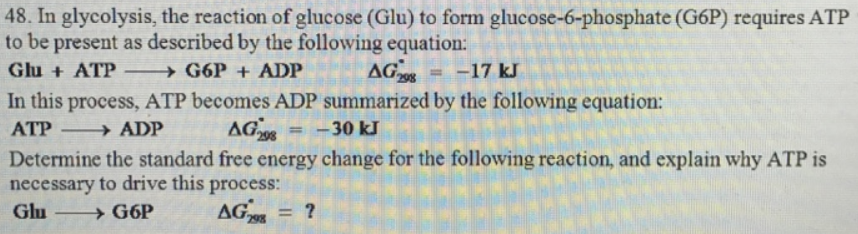# Problem: In glycolysis, the reaction of glucose (Glu) to form glucose-6-phosphate (G6P) requires ATP to be present as described by the following equation: Glu + ATP → G6P + ADP        ΔG°298 = -17 kJIn this process, ATP becomes ADP summarized by the following equation: ATP → ADP      ΔG°298 = -30 kJDetermine the standard free energy change for the following reaction, and explain why ATP is necessary to drive this process:

###### FREE Expert Solution
81% (326 ratings)###### Problem Details

In glycolysis, the reaction of glucose (Glu) to form glucose-6-phosphate (G6P) requires ATP to be present as described by the following equation:

Glu + ATP → G6P + ADP        ΔG°298 = -17 kJ

In this process, ATP becomes ADP summarized by the following equation:

ATP → ADP      ΔG°298 = -30 kJ

Determine the standard free energy change for the following reaction, and explain why ATP is necessary to drive this process: